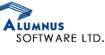# Placement Papers - AlumnusALUMNUS PAPER
Posted by :
NFDH
(22)
Hi Friends

There were 25 questions in all and all were Objective Type. Each question had 5 options.

1 When compiled from command line what are linking options?

2 You have written a code in C++, and u have to use a C library, what would u do?
(Ans: write extern "C" in header files)

3 Fiber optic backbone is in which OSI layer?

4 void main()
{
int x=1;
int y=1;
int i;
for(i=2;i<=100;i++)
{
x=x+i;y=y*(i+1)/(i-1);
}
}
What are the values of x & y?

5 If we carry out operation (-3)+(-6), then which of the what will be the value of carry and sign flag?

6 void abc(int a[])
{
int k=0;int j=50;
while(k<j)
{
if(a[i]>a[j])
k++;
else
j--;
}
}
How many times the loop will occur?

7 Integrate e^(x^-2)dx, with limits -infinity to +infinity? What is the final value?

8 Let p be a 16 bit number. The 2\'s complement of p will be represented by?

9 void main()
{
int a[]={5,4,3,2,1};
int x,y;
int *p=&a;
*p++;
x=++*p;
y=*(p++);
printf("%d %d", x, y);
}
What will be the value of x and y?

10 Let there be a set of 3 numbers. Then number of groups possible?

11 A question on some technique used in DA-converter?

12 Which data structure to use for fastest search?

13 A binary tree contains 1024 elements. What is maximum number of comparisons needed to search an element?

14 s=1-1/4+1/16-1/32... What is the value of S? (Ans = 0.8 Hint Its a GP)

15 A C++ class has multiple references to base class. Then some options were given?

16 for(i=0;i<20;i++)
{
a[i]=i;
}
for(i=0;i<20;i++)
{
a[i]=a[19-i];
}
What is final value of array a? Options were there.

17 In Java can a variable be initilised inside a loop?

18 Two dices are thrown. What is the probality that the the number on the first dice is greater or equal to number on the second dice?